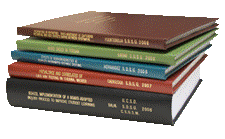## Libraries at University of Nebraska-Lincoln## Archival Master’s Theses from the University of Nebraska-Lincoln

Thesis

5-1963

#### Citation

Thesis (M.S.)—University of Nebraska—Lincoln, 1963. Department of Mathematics.

Copyright 1963, the author. Used by permission.

#### Abstract

One of the goals for any logic is to systematize and codify principles of valid reasoning.Mathematical logic may be considered as an extension of the formal method of mathematics to the field of logic; it employs for logic a symbolic language similar to that used in mathematics to express mathematical relations.A symbolic language of precisely defined character is necessary to avoid the ambiguity of the ordinary language.To achieve an exact scientific treatment of the subject we shall need clearly prescribed rules underlying reasoning processes.Therefore logical thinking will be reflected in a logical calculus.

The purpose of this thesis is to describe a logical calculus (the calculus of natural deduction) which characterizes predicate logic in the sense that every deducibility relation of the calculus is a consequence relation (the “soundness” of the calculus) and conversely, every consequence relation is a deducibility relation (the “completeness” of the calculus).

This paper gives a detailed account of ideas developed in the course in mathematical logic in 1962 by Dr. H.H. Schneider.The theorems appearing in this thesis which are stated without proof are established in the notes for the course in mathematical logic.